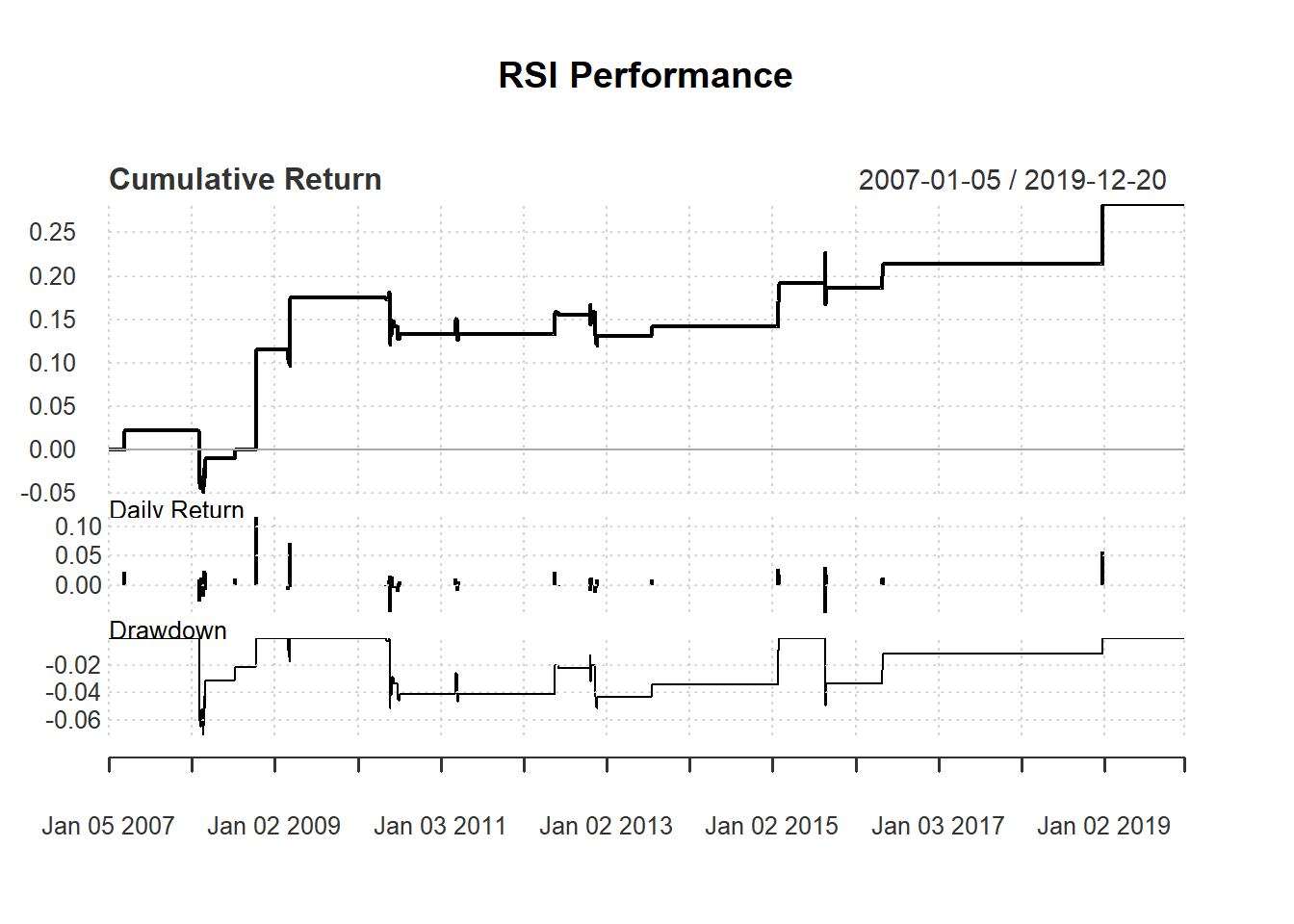## 7.4 Buy rule based on RSI

Consider following day-trading strategy based on 14-day RSI:

• buy one unit if RSI <30 and

Evaluate this based on day trading:

day <-14
price <- Cl(MSFT)
returnMSFT <- (Cl(MSFT) - Op(MSFT))/Op(MSFT)

signal <- c()                    #initialize vector
rsi <- RSI(price, day)     #rsi is the lag of RSI
signal [1:day+1] <- 0            #0 because no signal until day+1

for (i in (day+1): length(price)){
if (rsi[i] < 30){             #buy if rsi < 30
signal[i] <- 1
}else {                       #no trade all if rsi > 30
signal[i] <- 0
}
}
signal<-reclass(signal,Cl(MSFT))

To keep thing simple, we allow costless short selling. Otherwise, we only allow a sell after a buy.

trade <- Lag(signal)
charts.PerformanceSummary(ret)